# The relationship between information, bandwidth and noise

The most important question associated with a communication channel is the maximum rate at which it can transfer information. Information can only be transferred by a signal if the signal is permitted to change. Analog signals passing through physical channels may not change arbitrarily fast. The rate at which a signal may change is determined by the bandwidth. In fact the same Nyquist-Shannon law as governs the minimum sampling rate governs this rate of change; a signal of bandwidth B may change at a maximum rate of 2B. If each change is used to signify a bit, the maximum information rate is 2B.

The Nyquist-Shannon theorem makes no observation concerning the magnitude of the change. If changes of differing magnitude are each associated with a separate bit, the information rate may be increased. Thus, if each time the signal changes, it can take one of n levels, the information rate is increased to: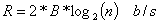This formula states that as n tends to infinity, so does the information rate.

Is there a limit on the number of levels? The limit is set by the presence of noise. If we continue to subdivide the magnitude of the changes into ever decreasing intervals, we reach a point where we cannot distinguish the individual levels because of the presence of noise. Noise therefore places a limit on the maximum rate at which we can transfer information. Obviously, what really matters is the signal-to-noise ratio (SNR). This is defined by the ratio of signal power S to noise power N, and is often expressed in decibels;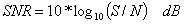Also note that it is common to see following expressions for power in many texts: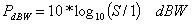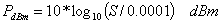i.e. the first equation expresses power as a ratio to 1 Watt and the second equation expresses power as a ratio to 1 milliWatt. These are expressions of power and should not be confused with SNR.

There is a theoretical maximum to the rate at which information passes error free over the channel. This maximum is called the channel capacity C. The famous Hartley-Shannon Law states that the channel capacity C is given by: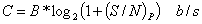Note that (S/N)P is the linear (not logarithmic) power ratio and B is the channel bandwidth (in cycles/second or Hertz) in this expression.  A more satisfying approximation to this equation is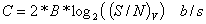Where the 2*B gives the maximum independent sample rate the channel can carry and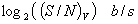is the number of bits required to describe the average number of discernable signal voltage levels given the strength of the noise.

For example, a standard telephone channel passes signals from 300 Hz to 3300 Hz yielding a 3 KHz Bandwidth (this BW limit is set by filters at the telephone office, not by the wire itself). It typically has a SNR of 40dB. The theoretical maximum information rate is then: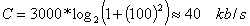Note that power is proportional to voltage squared.

The Hartley-Shannon Law makes no statement as to how the channel capacity is achieved. In fact, channels only approach this limit. The task of providing high channel efficiency is the goal of coding techniques. The failure to meet perfect performance is measured by the bit-error-rate (BER). Typically BERs are of the order of 10-6.